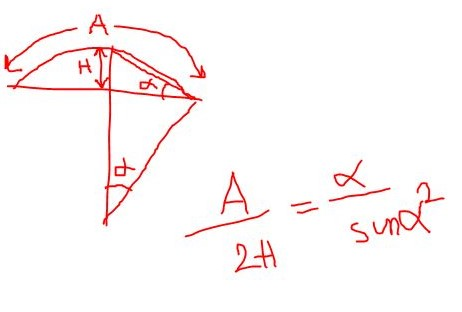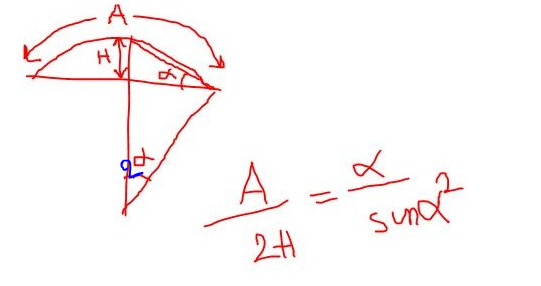# Arc based on start, end, radius

Hi everyone,

Is there any component in Grasshopper that draws an arc based on start point, end point and the radius?
And if not, do you have any suggestions?
thanks in advanceMost of the time simple thinks in Rhino have no counterpart in Grasshopper has in Grasshopper there is no user interaction (choice of a view, mouse movement …).
The best for you is to make a component from Arc SED (2 points and a vector) and calculate the vector depending on the plane, direction you want and radius.
Or use this plugin

1 Like

Use math to find the point if you choose start,end,radius or vector if you choose arc sed.
Of course a component to do that is better.

arc.gh (18.2 KB)

1 Like

How do you know it’s the short arc instead of the long one? What if you want that arc to be in a different plane?

I don’t understand what you mean by short and long arc.
It’s based on 2 points.

I wrote an algorithm, I know it’s not so clean and the shortest way, but for me it works…
But I can’t understand why the curve doesn’t shatter…ARC,Start,End,Radius.gh (18.3 KB)

An arc is part of a circle. So when you cut a circle at two points, you get two arcs. So even in 2d, two points and a radius actually describe two different circles and thus four different arcs. How do you pick between these four outcomes?

``````private void RunScript(Plane plane, Point3d start, Point3d end, double radius, ref object A, ref object B, ref object C, ref object D)
{
var line = new Line(start, end);
var vec = Vector3d.CrossProduct(line.Direction, plane.ZAxis);
vec.Unitize();
var d = Math.Sqrt(Math.Pow(radius, 2.0) - Math.Pow(line.Length, 2.0) / 4.0);
A = new Arc(start, line.PointAt(0.5) + vec * (radius - d), end);
B = new Arc(start, line.PointAt(0.5) - vec * (radius - d), end);
C = new Arc(start, line.PointAt(0.5) + vec * (radius + d), end);
D = new Arc(start, line.PointAt(0.5) - vec * (radius + d), end);
}
``````

Arc.gh (5.9 KB)

3 Likes

I don’t cut a circle, i draw a line between two points than offset its middle point , the maximum offset is the Radius.
There is a mathematical relation between the middle point of arc and the radius which used to create it.

1 Like

This is a python component to create arc from 2 points and Radius

arc SER.ghpy (31.5 KB)

``````import rhinoscriptsyntax as rs
import math

if S and E:
V = rs.VectorCreate(S,E)
L = rs.VectorLength(V)

if R < L/2 or R == None:
R = L/2

if D == True:
if A == True:
ang = math.degrees(math.asin(L/(2*R)))
else:
ang = math.degrees(-math.asin(L/(2*R)))

else:
if A == True:
ang = math.degrees(math.asin(L/(2*R))-math.pi)
else:
ang = math.degrees(math.pi-math.asin(L/(2*R)))

d = rs.VectorRotate(V,ang,[0,0,1])
C = rs.ArcCenterPoint(Arc)
``````
2 Likes

Great as always,
thanks so much.

Hi, I try to use this function in my Grasshopper. I struggle to get an Arc output.Any ideas what went wrong?

I can’t know without the file

This is the file. The left part makes problems. The right part was simply to double check the functionality.

ArcPPR.gh (11.7 KB)

It is a problem of output, you can fix it like that

ArcPPR_.gh (10.9 KB)

Thank you, that solved the issue.

Calculate ARC distance based on only 2 constraints (Arc Lenght and Height)
Hi @Mahdiyar, nice approach! Is there any way to solve this geometry problem via quick iterations in
c#, I tried with Galapagos but was too slow to solve it. Any help I’d really appreciate it.
T.

ARC_distance_solver.gh (13.0 KB)

Using math we find this equation: `a-(0.5*L*sin(a)²/H) = 0`
` (L is A)`Than we create graph and find the intersection with x axis, we choose the second solution (the first one is always = 0)

ARC_distance_solver.gh (15.9 KB)

thanks, @seghierkhaled nice math version. I changed your GH native version (take the second intersection value instead of the last one). could you help me to fix your python version to take the same value to prevent the shortest “Chord”?
could you graphically explain to me the Arc angle and Radius calculation, please?

Why when I open a definition that has a python code take 3s -4s on average. I said any python in general, but when I recompute decrease to 15ms? why does that happen?

Thank you again!
T.

You need to use the condition Chord > Sagitta (H) , this is a fixed version

I don’t understand your question? if you mean how i found the equation

Using equality you can find the final equation

(sorry i made mistake in the posted image before , 2a instead of a)ARC_distance_solver_fixed.gh (17.6 KB)